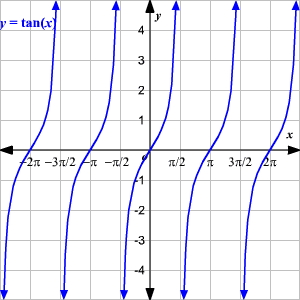# Graphing Tangent Function

The trigonometric ratios can also be considered as functions of a variable which is the measure of an angle. This angle measure can either be given in degrees or radians . Here, we will use radians.

Since, $\mathrm{tan}\left(x\right)=\frac{\mathrm{sin}\left(x\right)}{\mathrm{cos}\left(x\right)}$ the tangent function is undefined when $\mathrm{cos}\left(x\right)=0$ . Therefore, the tangent function has a vertical asymptote whenever $\mathrm{cos}\left(x\right)=0$ .

Similarly, the tangent and sine functions each have zeros at integer multiples of $\pi$ because $\mathrm{tan}\left(x\right)=0$ when $\mathrm{sin}\left(x\right)=0$ .

The graph of a tangent function $y=\mathrm{tan}\left(x\right)$ is looks like this:### Properties of the Tangent Function, $y=\mathrm{tan}\left(x\right)$ .

Domain : $x\in ℝ,\text{\hspace{0.17em}}\text{\hspace{0.17em}}x\ne \frac{\pi }{2}+n\pi$ , where $n$ is an integer.

Range : $\left(-\infty ,\infty \right)$

$y$ -intercept : $\left(0,0\right)$

$x$ -intercept : $n\pi$ , where $n$ is an integer.

Period: $\pi$

Symmetry: origin (odd function)

### Amplitude and Period of a Tangent Function

The tangent function does not have an amplitude because it has no maximum or minimum value.

The period of a tangent function, $y=a\mathrm{tan}\left(bx\right)$ , is the distance between any two consecutive vertical asymptotes.

Period = $\frac{\pi }{|\text{\hspace{0.17em}}b\text{\hspace{0.17em}}|}$

Also see Trigonometric Functions .